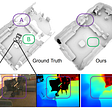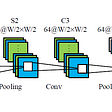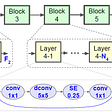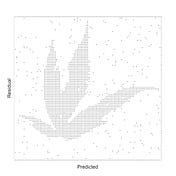# Use R to tackle Machine Learning (Andrew Ng) Course Homework-ex1

Grasping machine learning skills can be achieved by different tools. Concepts come from textbooks, online courses, or offline lectures, and practical skills by programming. Andrew Ng’s machine learning courses have well-organized tasks for practice, but the answers were written by Octave/Matlab. The series of article will guide you to reproduce Andrew Ng’s homework with R

# 0. Introduction

Hw1 is composed of (1) Basic function (2) Gradient Descent (3) Data visualization. The aim is to understand the principle of gradient descent, and the influences of parameter adjustment towards it. Then using simple plots helps learners interpret the concepts. By the way, as reading this article please go with ex1.pdf (Machine Learning Andrew Ng Courses hw1) for a better understanding.

# 1. Simple Octave/MATLAB(R) function

Return a 5x5 identity matrix. In R, it is just the short of Diagonal. See below:

`#### 5x5 identity matrix ####diag(5)`

# 2. Linear regression with one variable

The stories in the assignment would not intrigue you. You only need to know what to code for. Here is the classification:

— — Description Omitted — —

1. show the number of training data
2. draw a scatterplot of market size. X-axis: Population of City; Y-axis: Profit
3. set parameters of gradient descent(iterations, learning rate α, initial θ)
4. compute cost while setting different θ
5. run gradient descent, print θ for each iteration until the end
6. predict values for population sizes of 35,000 and 70,000
7. draw the regression line in the plot used θ
8. draw surface(3-D) and add processing line on it
9. draw contour(2-D) plots and point out the lowest cost dot

After classifying all the questions, the first priority is loading in the data and packages.

`#### packages loading ####install.packages("readr")   #for data read-ininstall.packages("ggplot2") #for data visualizationinstall.packages("rgl")     #for 3-D plottinginstall.packages("tidyr")   #Tools to tidy messy data#### data loading ####library(readr)data = read.delim("ex1data1.txt",header = F,sep = ",")x = data[,1] y = data[,2]`

2.1 Show the number of training data

`m = length(y) #or m = length(x)`

2.2 Draw a scatterplot of market size

`#### scatterplot of market size ####library(ggplot2)ggplot(data,aes(x = x,y= y))+  geom_point(colour = "red", shape = 4)+  labs(y = "Profit in \$10,000s",       x = "Population of City in 10,00s")`

2.3 Set parameters of gradient descent

`#### dim X matrix, iterations, learning rate α, initial θ####X = as.matrix(data.frame(rep(1,length(y)),x))theta= matrix(c(0,0),nrow=1)iterations = 1500alpha = 0.01`

2.4 Compute cost while setting different θ

In this session, we need to first build a handwritten function for computing costs. Next, the different θ should be set as a matrix to fit in the cost-computing function.

`#### cost computing function ####costcompute = function(theta){  X.matrix = X  theta= theta # cost function  cost = (1/(2*m)) *sum((t(y) - theta %*% t(X.matrix))^2)\  return(cost)}#### θ = [0,0] ####costcompute(theta = matrix(c(0,0),nrow=1))#### θ = [-1,2] ####costcompute(theta = matrix(c(-1,2),nrow=1))`

This task is the most principal in this assignment. Similar to 2.4, we have to build a gradient descent function. The function can be divided into small parts for easier understanding.

(1) Define X matrix, training number, and initial θ (In the previous session some of them have already set, but for the entirety, we would do it again inside the function).

(3) Write a loop to adjust θ and print the θ value in each iteration

`#### Built graient descent function ####gradientDescent = function(y, X, alpha, iters){## (1) Define X matrix, training number, and initial theta ##  X = as.matrix(data.frame(rep(1,length(y)),X))  m = length(y)## hand-write a gradient function to adjust θ ##  theta.init = matrix(c(0,0),nrow=1) # Initialize θ  theta = c(theta,theta)  theta = c(theta.init - alpha*(1/m)*sum((theta.init%*%t(X) - y)),            theta.init - alpha*(1/m)*(theta.init%*%t(X) - y ) %*% x)## Adjust θ and print the θ value in each iteration ##  cost = c()  print("theta0   theta1")  for(i in 1:iters){    cost_temp = (1/(2*m)) *sum((t(y) - theta%*%t(X))^2)    cost = c(cost,cost_temp)    grad = -(2/N) %*% (t(y) - theta%*%t(X)) %*% X    theta = c(theta - alpha*(1/m)*sum((theta%*%t(X) - y)),              theta - alpha*(1/m)*(theta%*%t(X) - y ) %*% x)    print(theta)  }  print("Expected theta values:")  print("theta0   theta1")  return(theta)}# print theta to screen by running functiongradientDescent(y = y, X = x, alpha = alpha, iters = iterations)`

2.6 Predict value for population sizes of 35,000 and 70,000

Because the original data omits 10,000, the prediction needs to multiple 10,000. θ is composed of θ0 and θ1, and they can be regarded as the slope(θ1) and the intercept(θ0) in the linear regression. This concept is also useful for drawing a line in the next task.

`#### Predict values ####theta = gradientDescent(y = y, X = x, alpha = alpha, iters =  iterations)predict1 = c(1,3.5) %*% theta * 10000predict2 = c(1,7) %*% theta * 10000`

2.7 Draw the regression line in the plot used θ

`#### draw the regression line in the plot used θ ####ggplot(data,aes(x = x,y= y))+  geom_point(colour = "red", shape = 4)+  labs(main = "MarkerSize")+  geom_abline(intercept = theta , slope = theta,colour = "blue") # theta is θ0 and theta is θ1`

2.8 Draw surface (3-D) and add processing line on it

The surface plot is meant to show in any combination of θ0 and θ1 (as x-axis and y-axis), the cost value (z-axis). Therefore, Using grids to express θ’s would be the proper way. We also can divide this task into 3 parts.

(1) Set grid of θ0 and θ1, and calculate the cost value

(2) Depict surface plot used data from (1)

(3) Stretch a line of our gradient descent movement (from high cost to the lowest cost in the previous practice)

`#### draw surface(3-D) and add processing line on it ###### Set grid of θ0 and θ1, and calculate the cost value ##theta0.val = seq(-10, 10, length.out=100) theta1.val = seq(-2, 4, length.out=100) theta.grid = expand.grid(theta0.val ,theta1.val)# initialize J_vals to a matrix of 0' (# not neccessary)J.vals = matrix(0,nrow = length(theta0.val), ncol = length(theta1.val))# Orgainze as a θ0 x θ1 matrixJ.vals = apply(theta.grid,1, costcompute) #compute cost for each θJ.vals = matrix(J.vals,nrow = length(theta0.val), ncol = length(theta1.val))## Depict surface plot ##library(rgl) #RGL is a 3D visualization device systemopen3d() # open a new window for 3-D plotnbcol = 100color = rev(rainbow(nbcol, start = 0/6, end = 4/6)) #set colorJ.vals.col  = cut(J.vals, nbcol)persp3d(theta0.val, theta1.val, J.vals,col = color[J.vals.col],        xlab=expression(theta_0),ylab=expression(theta_1),        zlab="Cost",main = "Gradient Descent")## Stretch a line of our gradient descent movement ### modify original gradient descent function to return all θ  theta.layout = function(y, X, alpha, iters){  X = as.matrix(data.frame(rep(1,length(y)),X))  m= length(y)  theta.init = matrix(c(0,0),nrow=1) # Initialize theta  theta = c(theta,theta)  theta = c(theta.init - alpha*(1/m)*sum((theta.init%*%t(X) - y)),            theta.init - alpha*(1/m)*(theta.init%*%t(X) - y ) %*% x)  theta.evolve = c()  for(i in 1:iters){    theta = c(theta - alpha*(1/m)*sum((theta%*%t(X) - y)),              theta - alpha*(1/m)*(theta%*%t(X) - y ) %*% x)    theta.evolve = rbind(theta.evolve,theta)  }  return(theta.evolve)}theta.all = theta.layout(y = y, X = x, alpha = alpha, iters = iterations) # run function to get all θ# add points, line together and show on graphpoints3d(theta.all[, 1], theta.all[, 2], theta.all+10,          col="white",size=3.5)lines3d(theta.all[, 1], theta.all[, 2], theta.all+10, col="red")`

2.9 Draw contour(2-D) plots and point out the lowest cost dot

The contour plot possesses a similar function as a surface plot (the top view of the surface plot. Hence, we inherit the grid of θ and design a 20 contours spaced logarithmically between 0.01 and 100 (the reason for using a logarithmic scale is the contours are denser near the center). After that, the cross should be added at the lowest cost dot.

`#### draw contour(2-D) plots and point out the lowest cost dot ##### set space function, from 10^d1 to 10^d2 by nlogspace <- function(d1, d2, n){return(exp(log(10)*seq(d1, d2, length.out=n)))}# plot contour(theta0.val, theta1.val, J.vals, levels = logspace(-2, 3, 20),         xlab=expression(theta_0),        ylab=expression(theta_1),        drawlabels = FALSE,        col = colorRampPalette(c("dark red","blue","green"))(8))# point out the lowest cost dot used red crosspoints(theta, theta, pch=4, cex=0.5,col="red",lwd=1.5)`

# 3. Linear regression with one variable

This session is an optional exercise, it is not mandatory to do. Nevertheless, as a
diligent student like you and me, we will manage to do it, right?

The stories in the assignment would not intrigue you. You only need to know what to code for. Here is the classification:

— — Description Omitted — —

1. standardize/normalize the dataset
2. implement the cost function and gradient descent for linear regression with multiple variables
3. draw a line graph about the convergence of cost
4. comparing learning rates by visualizing the convergence of descending cost with different learning rates (α)
5. predict value for price of a house using house sizes (1650 square feet) and the number of bedrooms (3 bedrooms).
6. use Normal Equations to do the same prediction

3.1 Standardize/normalize the dataset

`#### standardize/normalize the dataset ###### loadind datadata2 = read.delim("ex1data2.txt",header = F,sep = ",") # read comma separated data## standardize/normalize data2 as norm.data2norm.data2 = scale(data2)`

3.2 Implement the cost function and gradient descent and draw a line graph about the convergence of cost

The cost function is basically the same as 2.4, so you can just apply the function in this task. However, this task is also easier to interpret by dividing it into small parts.

(1) Define explanatory variables (x) and the response variable (y), X matrix, training number, initial θ, iterations, and learning rate given by assignment

(2) Introduce the cost function, gradient function to calculate the cost for initial θ and subsequent θs.

`#### implement the cost function and gradient descent ###### Define parameters ## x = norm.data2[,1:2]y = norm.data2[,3]m = length(y)X = as.matrix(data.frame(rep(1,length(y)),x))theta= matrix(c(0,0,0),nrow=1)iterations = 50alpha = 0.05## cost compute ##costcompute(theta=theta)## gradient function for calculating all cost value sequentiallygd.costlayout = function(y, X, alpha, iters){  X = as.matrix(data.frame(rep(1,length(y)),X))  m= length(y)  theta.init = matrix(c(1,2,3),nrow=1) # Initialize theta  theta = c(theta.init - alpha*(1/m)*sum((theta.init%*%t(X) - y)),            theta.init - alpha*(1/m)*(theta.init%*%t(X) - y ) %*% x[,1],            theta.init - alpha*(1/m)*(theta.init%*%t(X) - y ) %*% x[,2])  cost = (1/(2*m)) *sum((t(y) - theta.init%*%t(X))^2)  all.theta = c()  for(i in 1:iters){    cost_temp = (1/(2*m)) *sum((t(y) - theta%*%t(X))^2)    cost = c(cost,cost_temp)    grad = -(2/m) %*% (t(y) - theta%*%t(X)) %*% X    theta = c(theta - alpha*(1/m)*sum((theta%*%t(X) - y)),              theta - alpha*(1/m)*(theta%*%t(X) - y ) %*% x[,1],              theta - alpha*(1/m)*(theta%*%t(X) - y ) %*% x[,2])  }  return(cost)}# print cost to screen by running functioncost.layout(y = y, X = x, alpha = alpha, iters = iterations)`

3.3 Draw a line graph about the convergence of cost

`#### line graph about the convergence of cost ####dat.cost = data.frame(cost = all.cost, iters = 0:iterations)ggplot(data = dat.cost, aes(x = iters, y = cost))+  geom_line(group=1,col="green")+  labs(x = "number of literations", y = "Cost J")`

3.4 Comparing learning rates by visualizing the convergence of descending cost with different learning rates (α)

`#### learning rate comparison by line graph visualizing #####calculate all cost with different learning ratesdat.alpha = data.frame(alpha_0.01 = cost.layout(y = y, X = x, alpha = 0.01, iters = iterations),alpha_0.05 = cost.layout(y = y, X = x, alpha = 0.05, iters = iterations),alpha_0.1 = cost.layout(y = y, X = x, alpha = 0.1, iters = iterations),iters = 0:iterations)library(tidyr)dat.cost = dat.alpha %>% gather(.,"label","cost",-iters) # tidy to readable data frame for ggplotggplot(data = dat.cost, aes(x = iters, y = cost,color = label))+  geom_line()+  labs(x = "number of literations", y = "Cost J")`

3.5 Predict value for price of a house

The predicting equation is similar to variable regression. θ0 still represents intercept, and θ1, θ2 represent x1 slope and x slope respectively. However, in this task, we can easily accomplish by using matrix multiplication.

`#### Predict value ##### prediction with freature normalizationpredict3 = c(1,(1650-mean(data2\$V1))/sd(data2\$V1),(3-mean(data2\$V2))/sd(data2\$V2)) %*% theta#transform backpredict3 = predict3*sd(data2\$V3)+mean(data2\$V3)`

Note: because we predict with feature normalization, the answer needs to transform back to the actual value.

3.6 Use Normal Equations to do the same prediction

The normal equation is a substitution of gradient descent for θ calculation. The formula is below:

Leveraging on it, we only need to define the matrix of explanatory variables (X) and the response variable (y). Then θ and predicted value can be calculated.

`#### Use Normal Equations to do the same prediction ####data = read.delim("ex1data2.txt",header = F,sep = ",")X = as.matrix(cbind(1,data2[,1:2]))y = as.matrix(data2[,3])theta = (solve(t(X) %*% X ) %*% t(X)) %*% ypredict =  c(1,1650,3) %*%theta`

Reflection

After doing a big effort to absorb the concept and the complicated partial differential equation of Gradient Descent, the overwhelming Normal Equation hits straight ahead to me. In mandarin, we have an idiom to describe that, which is “小巫見大巫”. Nevertheless, the practice is definitely worthy. The basic knowledge of every discipline would be the principle cornerstone to go farther and further. Enjoy learning!

--

--

--

## More from AgriEnvCoder

Programmer | Agriculturist | Environmentalist

Love podcasts or audiobooks? Learn on the go with our new app.

## Introduction to Random Forest algorithm through song popularity prediction## Graph Representation Learning on Heterogeneous Graphs## Atlas — Neural Network Reconstructing a 3D Scene From Image 📸## Logistic Regression for an Advertising Company## Reading: AQ-CNN — Adaptive QP Convolutional Neural Network (Fast 3D-HEVC Prediction)## Review — Quo Vadis, Action Recognition? A New Model and the Kinetics Dataset (Video Classification)## [Paper] MnasNet: Platform-Aware Neural Architecture Search for Mobile (Image Classification)## Support Vector Machine (SVM) Hyperparameter Tuning In Python## AgriEnvCoder

Programmer | Agriculturist | Environmentalist

## Functions in R: how to get your output## Fast Data Science- utilmy## How to Understand Our Complex World with Data